|
|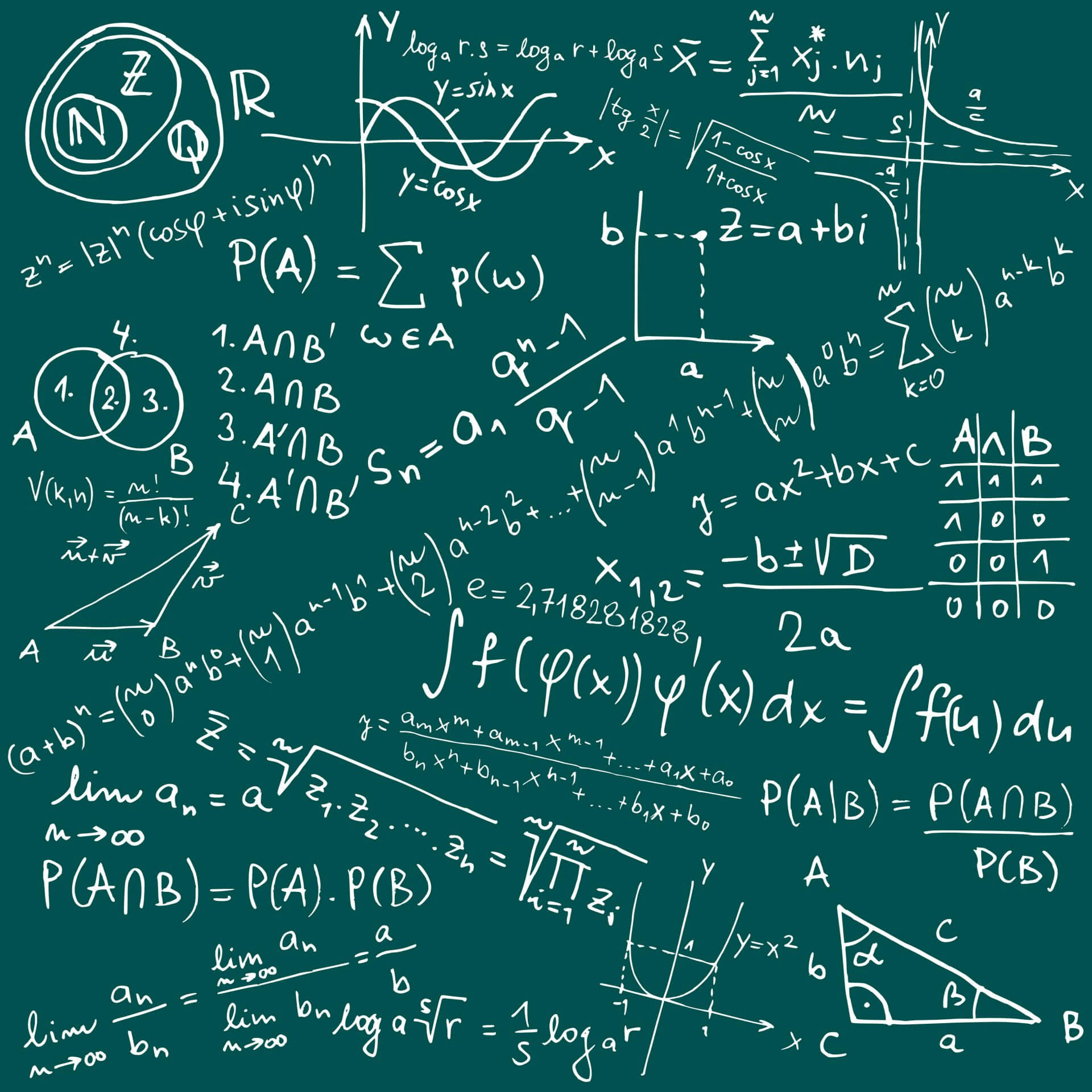### What are IIP3 and OIP3?

March 22, 2023

These two factors are used to characterize the linearity of a device, usually an amplifier. If two frequency signals (tones) are combined and amplified by a perfect amplifier, the output will simply be the same two tones with amplitudes increased by the gain. But amplifiers are not perfect, and always exhibit some degree of non-linearity.

An amplifier with a second order contribution will develop second harmonics of the two tones plus the sum and difference of the two tone frequencies. A third order contribution will develop intermodulation products on either side of the original two tones, equally spaced. If the input amplitude of both tones is increased by 1 dB, the output tones will increase by 1 dB and the two third order intermodulation products on either side will increase by 2 dB. If the two-tone input level is increased sufficiently, there is a theoretical output level where all four tones are the same amplitude. This level is called the third-order output intercept, or OIP3.

In practice, it is usually impossible to see all four tones at the same amplitude since gain compression usually sets in well before this can happen, but the theoretical level may be extrapolated from a measurement of the difference between the two main tones and the intermodulation products when they are at a much lower level, as shown in Figure 1.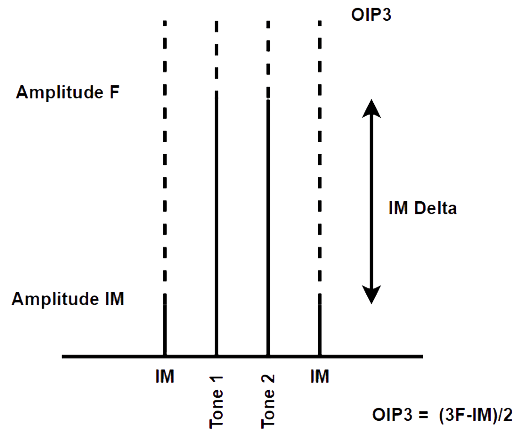Figure 1 – OIP3 Calculation

With knowledge of the OIP3 of an amplifier, you can calculate the level of the third-order intermodulation products for any given input level of two signals. This is important for determining the amount of interference created in adjacent channels in a communication system when two or more channels are active simultaneously.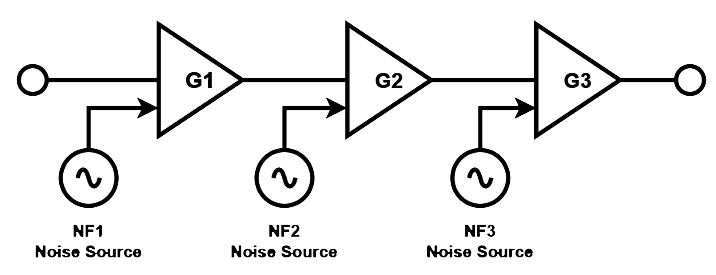Figure 2 – Amplifier Chain with Noise

The cumulative OIP3 of a chain of amplifiers like Figure 2 is given by: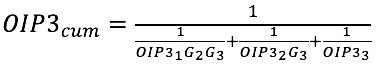Where the OIP3 and Gains are all converted to linear terms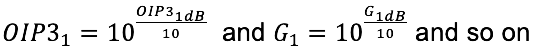Examining the formula shows that if the last stage has reasonable gain, then its OIP3 will dominate the cumulative system OIP3. The formula can also be expanded for any number of amplifiers.

The input IP3 or IIP3 is simply the OIP3 minus the gain of the amplifier. Mixers are generally specified with IIP3 while amplifiers are specified by OIP3.

See this and more important radio frequency charts and formulas in this comprehensive application note